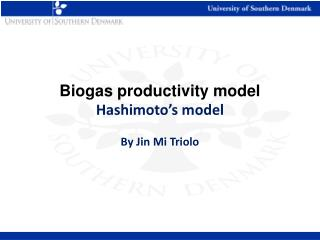Download PresentationBiogas productivity model Hashimoto’s model By Jin Mi Triolo

# Biogas productivity model Hashimoto’s model By Jin Mi Triolo

Download Presentation## Biogas productivity model Hashimoto’s model By Jin Mi Triolo

- - - - - - - - - - - - - - - - - - - - - - - - - - - E N D - - - - - - - - - - - - - - - - - - - - - - - - - - -
##### Presentation Transcript

1. Biogas productivity model Hashimoto’s model By Jin Mi Triolo

2. Hashimoto’s kinetic model γ = volumetric CH4 production rate, m3CH4 m-3 reactor volume per day, B0 =ultimate CH4 yield, m3CH4 kg-1(VS), S0 = influent VS concentration Kg(VS)/m3(feed) , feed is biomass μm = maximum specific growth rate/day, days-1, μm = 0.013T – 0.129 HTR= hydraulic retention times/ day, K=kinetic parameter, dimensionless (K=0.6+0.0206 exp (0.051*S0))

3. Hashimoto’s kinetic model • CH4 productivity is a function of • ultimate CH4 yield (B0) • influent substrate concentration(volatile solids, VS) • retention time (HTR) • kinetic parameters K • μm • K = 0.6+0.0206 exp (0.051*VS) • μm = 0.013T – 0.129 T = temperature For assessing biogas yield by Hashimoto’s model B0, VS, Operation time(HRT), operation temperature(T) Required !

4. Hashimoto’s two kinetic parameters K = 0.6+0.0206 exp (0.051*S0)) μm = 0.013T – 0.129 T = temperature Following Hashimoto, μm = temperature dependent μm increase linearly, as T increases But only in (20°C < T <60°C) μm is not applicable for the T lower than 20°C. Requirement of building new kinetic algorithm for lower temperatures. • When VS increases, • K increases exponentially. • Inhibition strength simply estimated only using VS concentration, but highly • predictible.

5. Example of hashimoto’s curve Batch experiment Predicted by Hashimoto’s model

6. Exercise • Pig fattening manure • 5 pigs • BMP :350 L/kg VS • Hydraulic retention time 30 d • Operation temperature 25 °C • Calculate how much slurry they excrete per day? • Calculate volumetric biogas yield at 25 °C? • Cattle manure • 2 cows • Calculate how much slurry they excrete per day • BMP :195 L/kg VS • Hydraulic retention time 30 d • Operation temperature 25 °C • Calculate how much slurry they excrete per day? • Calculate volumetric biogas yield at 25 °C?